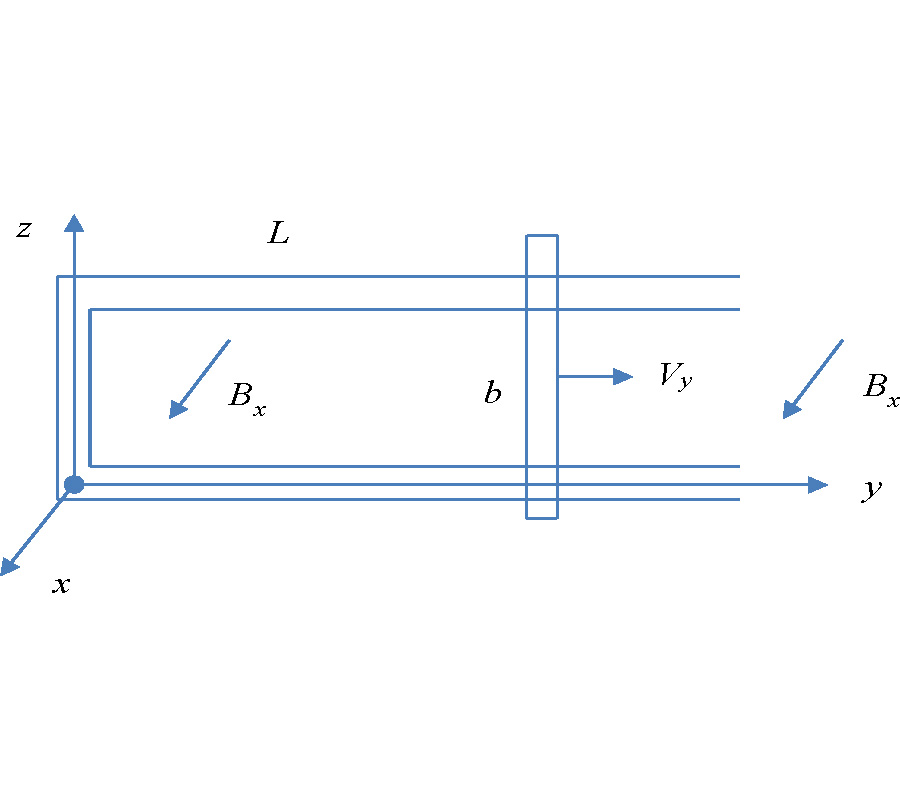# Vol. 96

Front:[PDF file] Back:[PDF file]
Latest Volume
All Volumes
All Issues
2019-10-01

#### On the Covariant Representation of Integral Equations of the Electromagnetic Field

By Sergey G. Fedosin
Progress In Electromagnetics Research C, Vol. 96, 109-122, 2019
doi:10.2528/PIERC19062902

## Abstract

Gauss integral theorems for electric and magnetic fields, Faraday's law of electromagnetic induction, magnetic field circulation theorem, theorems on the flux and circulation of vector potential, which are valid in curved spacetime, are presented in a covariant form. Covariant formulas for magnetic and electric fluxes, for electromotive force and circulation of the vector potential are provided. In particular, the electromotive force is expressed by a line integral over a closed curve, while in the integral, in addition to the vortex electric field strength, a determinant of the metric tensor also appears. Similarly, the magnetic flux is expressed by a surface integral from the product of magnetic field induction by the determinant of the metric tensor. A new physical quantity is introduced - the integral scalar potential, the rate of change of which over time determines the flux of vector potential through a closed surface. It is shown that the commonly used four-dimensional Kelvin-Stokes theorem does not allow one to deduce fully the integral laws of the electromagnetic field and in the covariant notation requires the addition of determinant of the metric tensor, besides the fact that the validity of the Kelvin-Stokes theorem is limited to the cases when determinant of metric tensor and the contour area are independent from time. This disadvantage is not present in the approach that uses the divergence theorem and equation for the dual electromagnetic field tensor. The problem of interpreting the law of electromagnetic induction and magnetic field circulation theorem cannot be solved on the basis of the Lorentz force in the absence of charges, and therefore requires a more general approach, when transformation of the field components from the reference frame at rest into the moving reference frame is taken into account. A new effect is predicted, according to which the circulation of magnetic field can appear even in the absence of electric current and with a constant electric field through the contour, if the area of this contour would change. By analogy with electromagnetic induction, for the magnetic field circulation to appear it is important that electric field flux passing through the area of the contour would change over time.

## Citation

Sergey G. Fedosin, "On the Covariant Representation of Integral Equations of the Electromagnetic Field," Progress In Electromagnetics Research C, Vol. 96, 109-122, 2019.
doi:10.2528/PIERC19062902
http://jpier.org/PIERC/pier.php?paper=19062902

## References

1. Feynman, R. P., R. B. Leighton, and M. L. Sands, The Feynman Lectures on Physics, Vol. 2, Pearson/Addison-Wesley, San Francisco, 2006, ISBN 0805390499.

2. Simonyi, K., Theoretische Elektrotechnik, 5th Ed., VEB Deutscher Verlag der Wissenschaften, Berlin, 1973.

3. Thorne, K. S. and D. Macdonald, "Electrodynamics in curved space-time: 3 + 1 formulation," Monthly Notices of the Royal Astronomical Society, Vol. 198, 339-343, 1982, https://doi.org/10.1093/mnras/198.2.339.
doi:10.1093/mnras/198.2.339

4. Tsagas, C. G., "Electromagnetic fields in curved spacetimes," Class. Quantum Grav., Vol. 22, No. 2, 393-407, 2005, https://doi.org/10.1088/0264-9381/22/2/011.
doi:10.1088/0264-9381/22/2/011

5. Cabral, F. and F. S. N. Lobo, "Electrodynamics and spacetime geometry: Foundations," Foundations of Physics, Vol. 47, No. 2, 208-228, 2017, http://dx.doi.org/10.1007/s10701-016-0051-6.
doi:10.1007/s10701-016-0051-6

6. Sorkin, R., "The quantum electromagnetic field in multiply connected space," Journal of Physics A: Mathematical and General, Vol. 12, No. 3, 403-422, 1979, https://doi.org/10.1088/0305-4470/12/3/016.
doi:10.1088/0305-4470/12/3/016

7. Landau, L. D. and E. M. Lifshitz, The Classical Theory of Field, Pergamon Press, 1951, ISBN7-5062-4256-7.

8. Dirac, P. A. M., General Theory of Relativity, Princeton University Press, 1975, ISBN 0-691-01146-X.